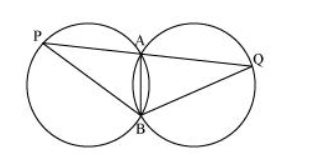# Two congruent circles intersect each other at points A and B.

Question. Two congruent circles intersect each other at points A and B. Through A any line segment PAQ is drawn so that P, Q lie on the two circles. Prove that BP = BQ.

Solution:$\mathrm{AB}$ is the common chord in both the congruent circles.

$\therefore \angle \mathrm{APB}=\angle \mathrm{AQB}$

In $\triangle B P Q$,

$\angle \mathrm{APB}=\angle \mathrm{AQB}$

$\therefore B Q=B P$ (Angles opposite to equal sides of a triangle)